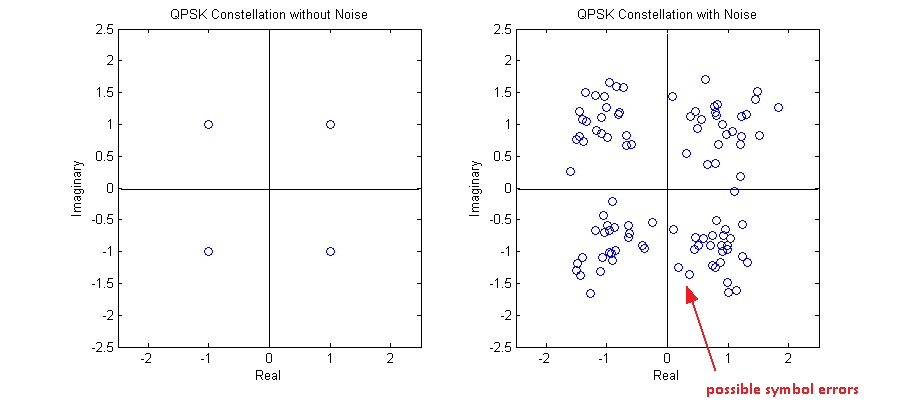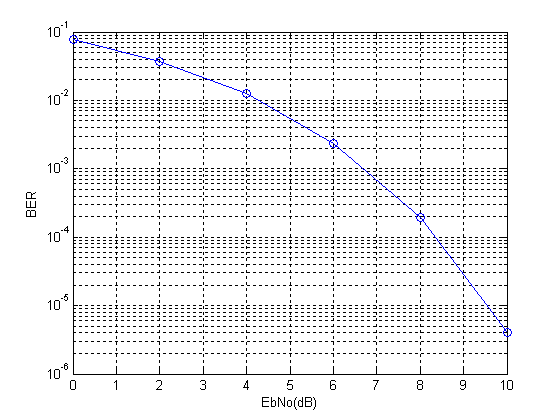# Bit Error Rate of QPSK

Simulating a QPSK system is equivalent to simulating two BPSK systems in parallel. So there is no difference in bit error rate(BER). Since the simulation is at baseband we multiply the in-phase and quadrature streams by 1 and j respectively (instead of cos and sin carriers). At the receiver we just use the real and imag functions to separate the two symbol streams. The BER is the average BER of the two parallel streams.

As in the case of BPSK we can show that the baseband representation (using 1 and j)  is equivalent to using the passband representation (using cosine and sine). Lets assume the following signal model for QPSK.

s(t)=a(t)*cos(2*pi*f*t)+b(t)*sin(2*pi*f*t)

where a(t) and b(t) contain the information to be transmitted over the channel. Now at the receiver we multiply this signal with cos( ) to recover a(t) and sin( ) to recover b(t).

cos(2*pi*f*t)*s(t)

=cos(2*pi*f*t) [a(t)*cos(2*pi*f*t)+b(t)*sin(2*pi*f*t)]

=a(t)*cos(2*pi*f*t)*cos(2*pi*f*t) +b(t)*sin(2*pi*f*t)*cos(2*pi*f*t)

=(a(t)/2)*(1+cos(4*pi*f*t))+(b(t)/2)*sin(4*pi*f*t)

=(a(t)/2)+(a(t)/2)*cos(4*pi*f*t)+(b(t)/2)*sin(4*pi*f*t)

After low pass filtering (LPF) we get (a(t)/2), which it the required in-phase component scaled by a constant 1/2. Similarly we can find the quadrature component b(t).

sin(2*pi*f*t)*s(t)

=sin(2*pi*f*t) [a(t)*cos(2*pi*f*t)+b(t)*sin(2*pi*f*t)]

=a(t)*sin(2*pi*f*t)*cos(2*pi*f*t)+b(t)*sin(2*pi*f*t)*sin(2*pi*f*t)

=(a(t)/2)*sin(4*pi*f*t)+(b(t)/2)*(1-cos(4*pi*f*t))

=(a(t)/2)*sin(4*pi*f*t)+(b(t)/2)-(b(t)/2)cos(4*pi*f*t)

Again after low pass filtering (LPF) we are left with the quadrature component b(t) scaled by the constant 1/2. So we can conclude that the multiplication by the carrier terms at the transmitter and receiver is not required in simulation and we can simply transmit a(t) and b(t). We just have to make sure that a(t) and b(t) are orthogonal to each other so that they do not interfere.

So the transmitted QPSK signal would have the form.

s(t)=a(t)+j*b(t)

The steps involved in the simulation are

1. Generate a random sequence of symbols for the in-phase and quadrature components (-1 corresponding to binary value of 0 and +1 corresponding to binary value of 1). Add the in-phase and quadrature components in the form a(t)+j*b(t).

2. Generate complex samples of Additive White Gaussian Noise (AWGN) with the required variance (noise power = noise variance OR noise power = square of noise standard deviation OR noise power = noise power spectral density * signal bandwidth).

3. Add AWGN samples to the QPSK signal.

4. Detection is performed at the receiver by determining the sign of the in-phase and quadrature components.

5. And finally the bit error rate (BER) is calculated for the in-phase and quadrature components. Total bit error rate is the mean of the two values.

```%%%%%%%%%%%%%%%%%%%%%%%%%%%%%%%%%%%%%%%%%%%%%%%%%%%%%%%%%%%%%%%%%%%%%%%%%%%%
% FUNCTION TO CALCULATE BER OF QPSK IN AWGN
% l - Input, length of the symbol sequence
% EbNo - Input, energy per bit to noise power spectral density
% ber - Output, bit error rate
%%%%%%%%%%%%%%%%%%%%%%%%%%%%%%%%%%%%%%%%%%%%%%%%%%%%%%%%%%%%%%%%%%%%%%%%%%%%
function[ber]=err_rate2(l,EbNo)
si=2*(round(rand(1,l))-0.5);               % In-phase component
s=si+j*sq;                              % QPSK symbol
n=(1/sqrt(2*10^(EbNo/10)))*(randn(1,l)+j*randn(1,l)); % AWGN Noise
r=s+n;                                  % Received signal with noise
si_=sign(real(r));                        % Detected in-phase component
ber1=(l-sum(si==si_))/l;                  % Bit error rate in-phase
ber2=(l-sum(sq==sq_))/l;                  % Bit error rate quadrature
ber=mean([ber1 ber2]);                    % Mean bit error rate
return
%%%%%%%%%%%%%%%%%%%%%%%%%%%%%%%%%%%%%%%%%%%%%%%%%%%%%%%%%%%%%%%%%%%%%%%%%%%%
```One final comment that I want to make is that bit error rate and symbol error rate is not always the same. Taking the example of QPSK a symbol error might occur when there is an error in the in-phase stream or the quadrature stream or both. So it is not a one to one mapping!!!

Note:

1. For a QPSK constellation centered around the origin of the co-ordinate system, the decision boundaries are simply defined by the x and y axes.

2. The reason for the name QPSK is that there are four symbols in the constellation, each having one of four possible phases (45, 135, 225, 315).#### Author: Yasir Ahmed (aka John)

More than 20 years of experience in various organizations in Pakistan, the USA, and Europe. Worked as a Research Assistant within the Mobile and Portable Radio Group (MPRG) of Virginia Tech and was one of the first researchers to propose Space Time Block Codes for eight transmit antennas. The collaboration with MPRG continued even after graduating with an MSEE degree and has resulted in 12 research publications and a book on Wireless Communications. Worked for Qualcomm USA as an Engineer with the key role of performance and conformance testing of UMTS modems. Qualcomm is the inventor of CDMA technology and owns patents critical to the 4G and 5G standards.

5.00 avg. rating (92% score) - 1 vote

## 3 thoughts on “Bit Error Rate of QPSK”

1.Yves says:

I this the theorical or experimental code ?

1.John (YA) says:

The graphs were generated through simulation in MATLAB…so experimental not theoretical…hope that answers your question.

2.mrigank bhaskar says: